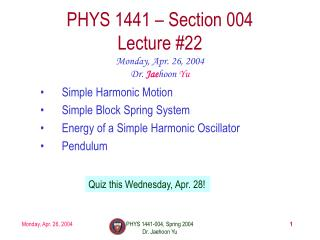Download PresentationPHYS 1441 – Section 004 Lecture #22

# PHYS 1441 – Section 004 Lecture #22 - PowerPoint PPT PresentationDownload Presentation## PHYS 1441 – Section 004 Lecture #22

- - - - - - - - - - - - - - - - - - - - - - - - - - - E N D - - - - - - - - - - - - - - - - - - - - - - - - - - -
##### Presentation Transcript

1. PHYS 1441 – Section 004Lecture #22 Monday, Apr. 26, 2004 Dr. Jaehoon Yu • Simple Harmonic Motion • Simple Block Spring System • Energy of a Simple Harmonic Oscillator • Pendulum Quiz this Wednesday, Apr. 28! PHYS 1441-004, Spring 2004 Dr. Jaehoon Yu

2. Announcements • Instructor Evaluation Today • Next quiz on Wednesday, Apr. 28 • Covers: ch. 10.4 – ch.11.2, including all that are covered in the lecture PHYS 1441-004, Spring 2004 Dr. Jaehoon Yu

3. The sign is negative, because the force resists against the change of length, directed toward the equilibrium position. Vibration or Oscillation What are the things that vibrate/oscillate? • Tuning fork • A pendulum • A car going over a bump • Building and bridges • The spider web with a prey So what is a vibration or oscillation? A periodic motion that repeats over the same path. A simplest case is a block attached at the end of a coil spring. When a spring is stretched from its equilibrium position by a length x, the force acting on the mass is Acceleration is proportional to displacement from the equilibrium Acceleration is opposite direction to displacement This system is doing a simple harmonic motion (SHM). PHYS 1441-004, Spring 2004 Dr. Jaehoon Yu

4. Vibration or Oscillation Properties The maximum displacement from the equilibrium is Amplitude One cycle of the oscillation The complete to-and-fro motion from an initial point Period of the motion, T The time it takes to complete one full cycle Unit? s Frequency of the motion, f The number of complete cycles per second Unit? s-1 Relationship between period and frequency? or PHYS 1441-004, Spring 2004 Dr. Jaehoon Yu

5. Vibration or Oscillation Properties • When is the force greatest? • When is the velocity greatest? • When is the acceleration greatest? • When is the potential energy greatest? • When is the kinetic energy greatest? Amplitude? A PHYS 1441-004, Spring 2004 Dr. Jaehoon Yu

6. Example 11-1 Car springs. When a family of four people with a total mass of 200kg step into their 1200kg car, the car’s springs compress 3.0cm. (a) What is the spring constant of the car’s spring, assuming they act as a single spring? (b) How far will the car lower if loaded with 300kg? (a) What is the force on the spring? From Hooke’s law (b) Now that we know the spring constant, we can solve for x in the force equation PHYS 1441-004, Spring 2004 Dr. Jaehoon Yu

7. Energy of the Simple Harmonic Oscillator How do you think the mechanical energy of the harmonic oscillator look without friction? Kinetic energy of a harmonic oscillator is The elastic potential energy stored in the spring Therefore the total mechanical energy of the harmonic oscillator is Total mechanical energy of a simple harmonic oscillator is proportional to the square of the amplitude. PHYS 1441-004, Spring 2004 Dr. Jaehoon Yu

8. KE/PE E=KE+PE=kA2/2 -A A Energy of the Simple Harmonic Oscillator cont’d Maximum KE is when PE=0 Maximum speed The speed at any given point of the oscillation x PHYS 1441-004, Spring 2004 Dr. Jaehoon Yu

9. Example 11-3 Spring calculations. A spring stretches 0.150m when a 0.300-kg mass is hung from it. The spring is then stretched an additional 0.100m from this equilibrium position and released. (a) Determine the spring constant. From Hooke’s law (b) Determine the amplitude of the oscillation. Since the spring was stretched 0.100m from equilibrium, and is given no initial speed, the amplitude is the same as the additional stretch. PHYS 1441-004, Spring 2004 Dr. Jaehoon Yu

10. Example cont’d (c) Determine the maximum velocity vmax. (d) Determine the magnitude of velocity, v, when the mass is 0.050m from equilibrium. (d) Determine the magnitude of the maximum acceleration of the mass. Maximum acceleration is at the point where the mass is stopped to return. Solve for a PHYS 1441-004, Spring 2004 Dr. Jaehoon Yu

11. Example for Energy of Simple Harmonic Oscillator A 0.500kg cube connected to a light spring for which the force constant is 20.0 N/m oscillates on a horizontal, frictionless track. a) Calculate the total energy of the system and the maximum speed of the cube if the amplitude of the motion is 3.00 cm. From the problem statement, A and k are The total energy of the cube is Maximum speed occurs when kinetic energy is the same as the total energy PHYS 1441-004, Spring 2004 Dr. Jaehoon Yu

12. Example for Energy of Simple Harmonic Oscillator b) What is the velocity of the cube when the displacement is 2.00 cm. velocity at any given displacement is c) Compute the kinetic and potential energies of the system when the displacement is 2.00 cm. Kinetic energy, KE Potential energy, PE PHYS 1441-004, Spring 2004 Dr. Jaehoon Yu